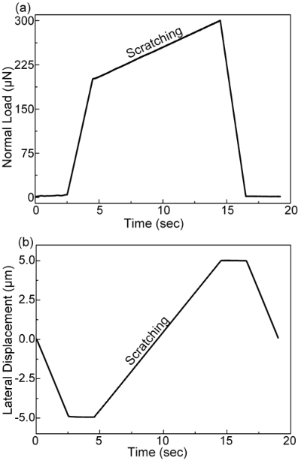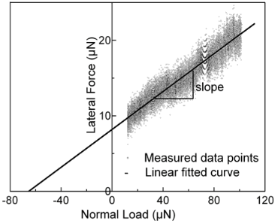# Effect of normal load and roughness on the nanoscale friction coefficient in the elastic and plastic contact regime

1. § ,
2. and
Institute of Materials Engineering, University of Siegen, Paul-Bonatz-Str. 9-11, 57076 Siegen, Germany
1. Corresponding author email
§ Tel. + 49 271 740 4065; Fax: + 49 271 740 2442
Guest Editors: P. Ziemann and T. Schimmel
Beilstein J. Nanotechnol. 2013, 4, 66–71. https://doi.org/10.3762/bjnano.4.7
Received 20 Nov 2012, Accepted 08 Jan 2013, Published 28 Jan 2013

## Abstract

The influence of applied normal load and roughness on the tribological behavior between the indenter and sample surface during nanoindentation-based scratching has been experimentally investigated by using different surfaces (fused silica and diamond-like carbon) featuring various degrees of roughness. At a sufficiently low applied normal load, wherein the contact is elastic, the friction coefficient is constant. However, at increased normal loads the contact involves plastic deformation and the friction coefficient increases with increasing normal load. The critical load range for a transition from predominantly elastic to plastic contact, between the indenter and sample surface, increases with increasing size of indenter and decreases with roughness. Distinct differences between the present experimental results and the existing theoretical models/predictions are discussed.

## Introduction

In this paper, various scratch tests with different linearly increasing normal loads for surfaces featuring different roughness values (fused silica (FS) and diamond-like carbon (DLC)) have been carried out. Aside from the normal load, the tip radius of the conical diamond indenter has been varied in these experiments. The friction coefficients were measured and compared to the GW and the KE model as well as the FEM-based model mentioned above. The goal was to study the effect of the applied normal load and roughness on the friction coefficient and the critical normal load regime for a transition from a predominantly elastic to a plastic contact between the indenter and surface of the sample during a nanoindentation-based scratch test with linearly increasing load.

## Experimental

Samples: As mentioned above, fused silica and DLC were chosen as sample materials. The fused silica was provided as a standard sample by Hysitron Inc. The DLC samples, 1µm thick films on Si(100) wafer, were synthesized by chemical vapor deposition (Balzer BAS 450) utilizing a gas mixture of argon and acetylene at a bias voltage of −950 and −350 V, respectively.

Topographical characterization: The surface morphology was characterized by atomic force microscopy (AFM, Park Systems Corp. XE-100). Noncontact AFM was used to obtain detailed information about surface topography and surface roughness. The samples were imaged with commercial tips featuring a nominal tip radius of 10 nm in a feedback-controlled mode on all three axes. Five 8 × 8 μm2 images with a pixel resolution of 512 × 512 were taken at different surface positions on each sample in order to derive the corresponding RMS roughness. The appropriate topography of the conical indenters utilized in the present work was also characterized. The resulting roughness of the 1 µm conical indenter was found to be negligible. The 20 µm conical indenter featured topography aside from the overall macroscopic conical one (with spherical end cap). However, its characteristic length scale was significantly larger than that of the samples studied here. For these reasons, we refrained from taking the indenter roughness into account in both cases.Figure 1: Example for a load (a) and displacement scheme (b) of one of the individual scratch segments used in this work. Figure 1: Example for a load (a) and displacement scheme (b) of one of the individual scratch segments used i... Jump to Figure 1Figure 2: Lateral force versus normal load plot for the fused silica sample in contact with the 1 µm conical indenter. Friction coefficient is estimated by a linear fitting routine. See text for details. Figure 2: Lateral force versus normal load plot for the fused silica sample in contact with the 1 µm conical ... Jump to Figure 2

## Results

The roughnesses along with the mechanical properties of the samples are given in Table 1. Analyzing the tribological data, some distinct differences in the behavior of the three samples that are the subject of this work are revealed. In order to take a detailed look at the behavior of the friction coefficient with respect to the applied normal load, the corresponding results have been plotted for all three samples and are shown in Figure 3, Figure 4, and Figure 5. These figures also show the error bars that were measured from multiple test data. The friction coefficient for all three samples was always higher for the 20 µm radius conical indenter than for the 1 µm radius conical indenter. Generally a low friction coefficient is observed at an early stage of each scratch, i.e., low applied normal loads. An increase of the normal load during scratching typically results in an increased coefficient of friction. This increase can be either continuous as in case of the rough DLC sample, see Figure 5, or the increase is found only if the normal load exceeds some certain critical load. The actual critical load of a transition from a low, apparently constant coefficient of friction to the linearly increasing one depends on the sample material and the roughness as well as the indenter used.

aPoisson ratio ν = 0.17; bν = 0.30Figure 3: Friction coefficient versus normal load for the fused silica sample derived with both conical indenters. The error bars show the standard deviation of data. Figure 3: Friction coefficient versus normal load for the fused silica sample derived with both conical inden... Jump to Figure 3Figure 4: Friction coefficient versus normal load for the smooth DLC sample derived for both conical indenters. The error bars show the standard deviation of the data. Figure 4: Friction coefficient versus normal load for the smooth DLC sample derived for both conical indenter... Jump to Figure 4Figure 5: Friction coefficient versus normal load for the rough DLC sample derived for both conical indenters. The error bars show the standard deviation of the data. Figure 5: Friction coefficient versus normal load for the rough DLC sample derived for both conical indenters... Jump to Figure 5

It is generally accepted that these transitions correspond to a transition from a predominantly elastic to a predominantly plastic contact between the sample and the indenter. For this reason it is obviously not possible to provide precise normal-load numbers for such a transition, as the contact between two rough surfaces will typically feature asperities that are deformed elastically along with those that are already plastically deformed. Table 2 (see below) gives the appropriate ranges of normal loads during scratching for which the above-mentioned transitions have been observed in the experiments carried out here. Silica as well as the smooth DLC sample shows a transition between a predominantly elastic and a predominantly plastic contact. The observed values here are 400–500 µN and 1800–2100 µN as well as 50–70 µN and 200–250 µN for tests carried out with the 1 µm and the 20 µm conical diamond indenter on silica (Figure 3) as well as on the smooth DLC sample (Figure 4), respectively. For the rough DLC sample any load regimes featuring a predominantly elastic contact were not identified. Therefore, no transition was observed and a predominantly plastic contact is established already at very low loads (Figure 5). It was also observed that the critical load range increases with increasing indenter size. These findings show that the combination of mechanical properties, sample roughness, and indenter radius are key parameters in determining the contact characteristics, i.e., whether the indenter is in a predominantly elastic or plastic contact, at a given normal load.

aGreenwood and Williamson model; bKogut and Etsion model.

## Discussion

In this work the tribological contact behaviors between two conical diamond indenters and fused silica as well as diamond-like carbon samples featuring different roughness during nanoindentation-based scratch test carried out with linearly increasing normal load were investigated. The friction coefficients were segmentally calculated from the slope of a linear fit to the lateral force versus normal load. The friction coefficient is found to increase with the size of indenter due to obvious reasons of increasing contact area, and hence the critical load regime will change accordingly. The results were compared with predictions by the GW as well as the KE model. In both cases the models estimate a predominantly elastic contact for the tests on fused silica and predominantly plastic contact for both DLC samples. This could not be verified by experiments as the fused silica sample showed a transition from predominantly elastic to plastic contact and the smooth DLC sample featured a predominantly elastic contact regime. Therefore, both observations are in contradiction to the models.

The general trend of a transition from a predominantly elastic contact regime featuring a low constant friction coefficient, to a predominantly plastic contact characterized by an increasing friction coefficient with increasing load, suggested by FEM calculations of Flores et al., was experimentally reproduced. However, the load boundaries predicted by the FEM model significantly overestimate the ones that were experimentally found. In addition to that, the FEM model fails to reproduce the significant differences between the two DLC samples of different roughness.

In summary the results presented here show that to date the existing contact models are not able to simulate the behavior of the friction coefficient during nanoindentation-based scratch tests. Especially the influence of sample roughness is not well understood. Therefore, the authors are in the process of carrying out a series of systematic tests on various samples featuring roughness variations allowing for a more detailed analysis of the effect of roughness on the load dependence of the coefficient of friction. The findings will be the content of a future publication.

## Conclusion

In this work, the influence of the applied normal load and roughness on the tribological behavior between the indenter and sample surface using a nanoindenter has been studied. The transition from a predominantly elastic contact regime featuring a constant coefficient of friction to a predominantly plastic contact characterized by an increasing coefficient of friction with increasing load was experimentally observed. It was found that the critical load range for a transition from predominantly elastic to plastic contact increases with increasing size of indenter and decreases with surface roughness. The experimental results were compared with the predictions of the model by Greenwood and Williamson and the one by Kogut and Etsion, as well as the FEM-based model by Flores et al. None of the presently available theoretical models was able to quantitatively describe the experimental results.

## Acknowledgements

The authors are indebted to Dr. R. Bandorf (Fraunhofer-Institut für Schicht- und Oberflächentechnik, Braunschweig) for providing the studied DLC materials.

Other Beilstein-Institut Open Science Activities